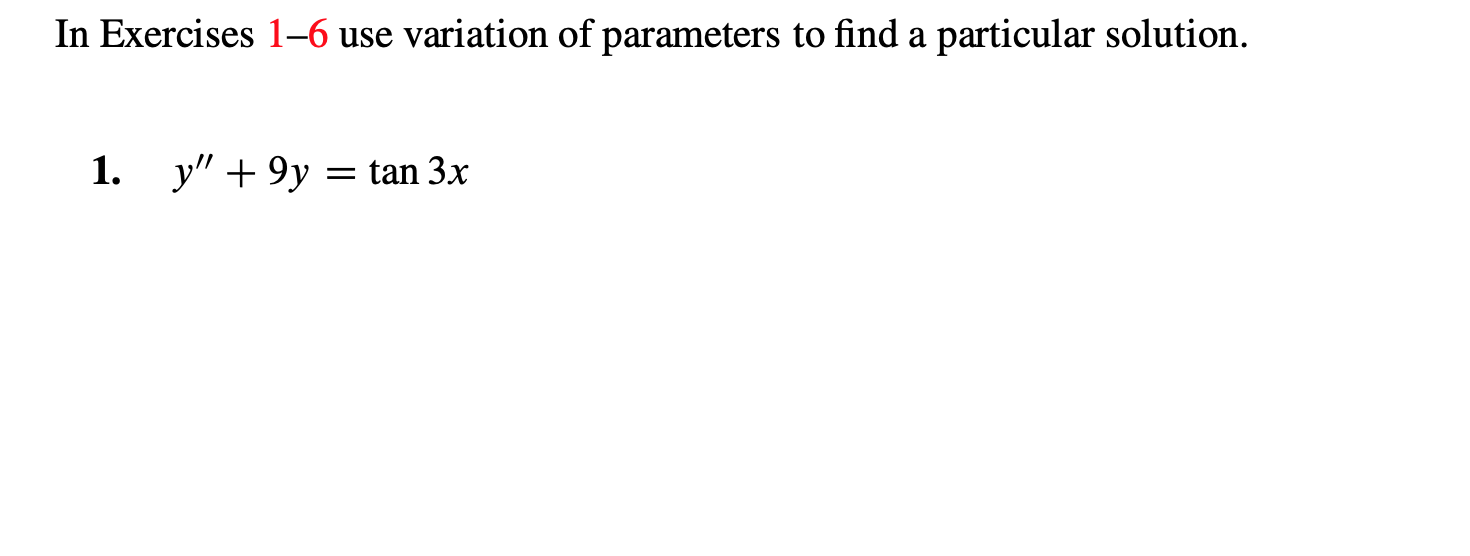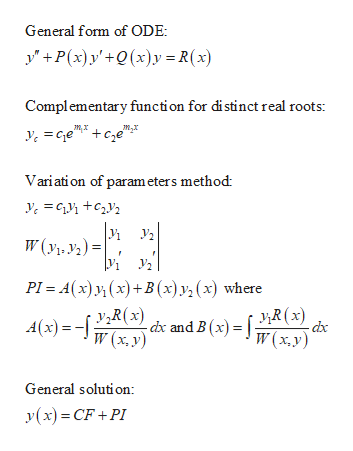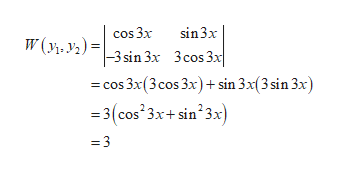# In Exercises 1-6 use variation of parameters to find a particular solution.y9y tan 3x1.

Questionhelp_outlineImage TranscriptioncloseIn Exercises 1-6 use variation of parameters to find a particular solution. y9y tan 3x 1. fullscreen
check_circleExpert Solution
Step 1

Result used:help_outlineImage TranscriptioncloseGeneral form of ODE P(x)y'+Q(x)y R(x) Complementary function for distinct real roots: mx m,x Variation of param eters method W(yi, y)= PI Ax)(x)+B (x)y (x) where y>R(x) dx and B (x) R(x) W(x.y) A (x)= W(x. y) General solution: y(x)CF+PI fullscreen
Step 2

The given differential equation is

Step 3

Find the complementary function as follows.

The auxiliary ...help_outlineImage Transcriptionclosecos 3x sin3x W(y, y2)= 3 sin 3x 3cos 3x cos 3x(3cos 3x) sin 3x(3 sin 3x) 3(cos 3x+ sin23x) =3 fullscreen

### Want to see the full answer?

See Solution

#### Want to see this answer and more?

Solutions are written by subject experts who are available 24/7. Questions are typically answered within 1 hour*

See Solution
*Response times may vary by subject and question
Tagged in

### Math